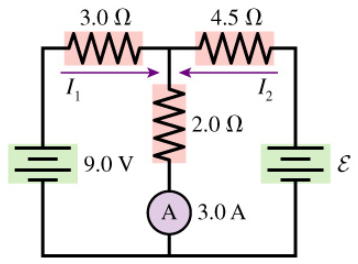# Problem: The ammeter in the figure reads 3.0 A.(a) Find I2. Express your answer using two significant figures, in Amps.(b) Find ε. Express your answer using two significant figures, in Volts.

###### FREE Expert Solution
97% (474 ratings)
###### FREE Expert Solution

The positive of a resistor is where the current enters the resistor. The negative of a resistor is where the current leaves the resistor. The longer terminal of a battery is positive while the shorter terminal is negative. When crossing an element from positive to negative, we use a negative sign. When crossing an element from negative to positive we use positive sign.

The voltage across a resistor, V= IR.

97% (474 ratings)###### Problem Details

The ammeter in the figure reads 3.0 A.
(a) Find I2. Express your answer using two significant figures, in Amps.
(b) Find ε. Express your answer using two significant figures, in Volts.